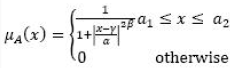# Artificial Intelligence - Fuzzy Sets

>>>>>>>>Fuzzy Sets

Let A be a generalized bell-shaped fuzzy number such thatand B = (b1, b2, b3) be a triangular fuzzy number.
State whether the following is TRUE or FALSE

• A

True• B

FalseLet A, B, C, D are closed intervals of R. Let A ⊆ C and B D, then state whether the following are TRUE or FALSE?

• A

True• B

FalseLet A, B, C, D are closed intervals of R. Let A ⊆ C and B D, then state whether the following are TRUE or FALSE?

• A

True• B

FalseLet A, B, C, D are closed intervals of R. Let A ⊆ C and B D, then state whether the following are TRUE or FALSE?

• A

True• B

FalseLet A, B, C, D are closed intervals of R. Let A ⊆ C and B D, then state whether the following are TRUE or FALSE?

• A

True• B

FalseRelated Quiz.
Fuzzy Sets